Worksheets and No Prep Teaching Resources
Critical Thinking Puzzles
Make Puzzles

# Sequences and Number Pattern Puzzles Puzzle Workbooks for Kids - Updated Each Month!

Several different critical thinking puzzles that relate to sequences, including find the sequence, pattern puzzles, and number sequences. Some of these are explicitly math related, such as finding the math pattern (these are excellent for test preparation), while others are great both for math teachers or any teachers, such as draw a sequence of pictures (great for younger kids in terms of teaching following directions). You also have the option of printing a mixed number pattern book for any grade, 3-6, or middle/high school. This page deserves to be explored with all of its sequencing options and customizations!
What better way to organize students' brains than by letting them have fun figuring out patterns and sequences, an important part of mathematics at all levels! These unusual worksheets are both beautifully designed and intricate, so students from second grade through high school have fun while learning to think logically and critically. Additional worksheets on skip counting, fraction and decimal sequencing, and even Roman numerals, make these worksheets invaluable for the classroom.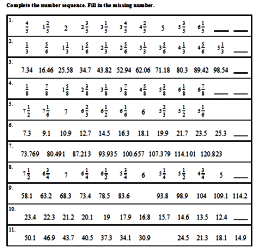Patterns

Pattern Puzzles - Find the Patterns and Color Puzzle

Sequencing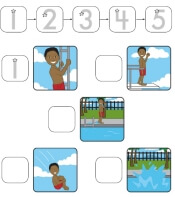Sequencing Book: Put numbers 1 to 5 next to pictures to complete the sequence

Patterns
Circle Growing or Decreasing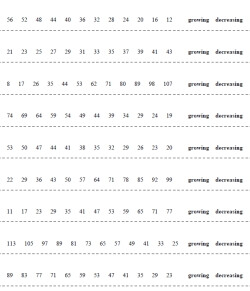Circle growing or decreasing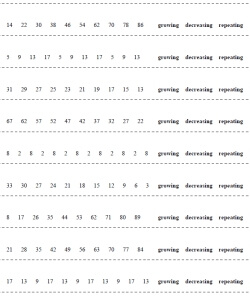Circle growing, decreasing, or repeating

Complete the Sequence - Change Boxes Given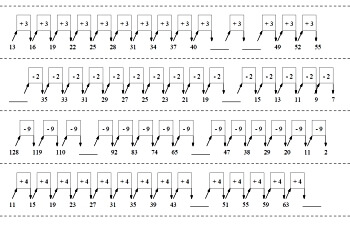Growing or Decreasing: Complete the pattern - all the change values are given - 2 blanks Growing or Decreasing: Complete the pattern - all the change values are given - 4 to 6 blanksGrowing, Decreasing, or Repeating: Complete the pattern - all the change values are given - 2 blanks Growing, Decreasing, or Repeating: Complete the pattern - all the change values are given - 4 to 6 blanks

Write the Rule - Change Boxes Given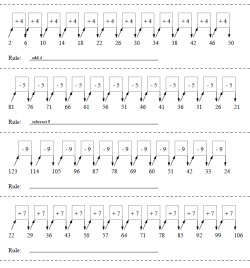Write the Rule: Growing or Decreasing (rule given for the first two problems) Write the Rule: Growing or Decreasing

Write the Rule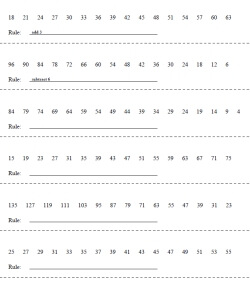Write the Rule: Growing or Decreasing (rule given for the first two problems)

Complete the Sequence and Fill-In Change Boxes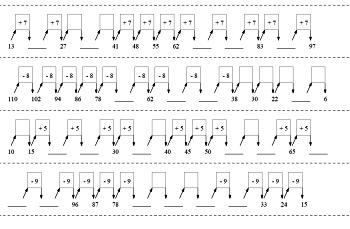Complete the pattern - 2 change values are blank - 2 blanks Complete the pattern - 2 to 4 change values are blank - 4 to 6 blanks

Complete the Sequence - Repeating Numbers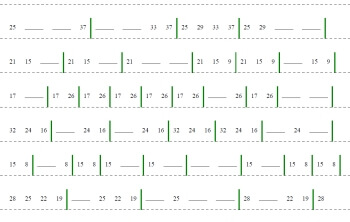Repeating: Complete the pattern - green lines to show repeating (2 to 4 blanks; landscape page) Repeating: Complete the pattern - green lines to show repeating (4 to 6 blanks; landscape page)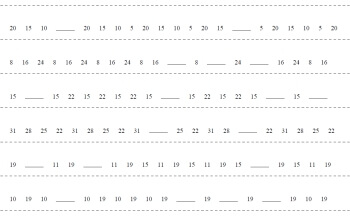Repeating: Complete the pattern (2 to 4 blanks; landscape page)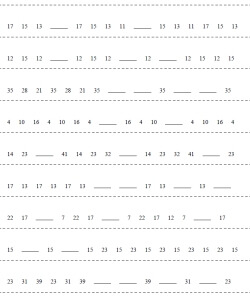Repeating: Complete the pattern (2 to 4 blanks) Challenging - Repeating: Complete the pattern (4 to 6 blanks)

Complete the Sequence - Growing or Decreasing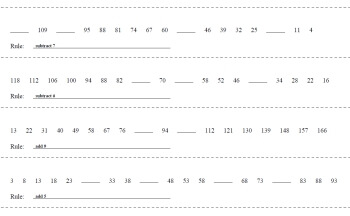Growing or Decreasing: Complete the pattern - Rule is Given (2 to 4 blanks; landscape page) Growing or Decreasing: Complete the pattern - Rule is Given (4 to 6 blanks; landscape page)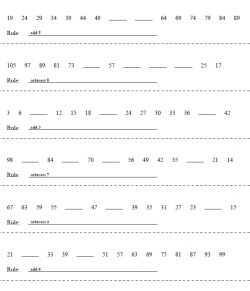Growing or Decreasing: Complete the pattern - Rule is Given (2 to 4 blanks) Growing or Decreasing: Complete the pattern - Rule is Given (4 to 6 blanks)

Number Sequence - Critical Thinking
Mixed Number Sequence Books
Number Pattern Book (middle school and high school)

Mixed number sequence printables
Easy mix of number sequences
Mix of decimal and fraction number sequences
Mix of whole number sequences
Mix of whole number sequences (more difficult)

Easy whole number sequences
Subtraction (add a fixed number from 1 to 9)
Multiplication (by 2..9)
Multiplication (by 2..12)
Division (by 2..9)
Division (by 2..12)
Multiplication and division (by 2..9)
Multiplication and division (by 2..12)

Roman numeral number sequences
Addition and subtraction using Roman numerals
Roman numeral addition sequences (some interwoven sequences)
Roman numeral subtraction sequences (some interwoven sequences)
Roman numeral addition and subtraction sequences (some interwoven sequences)

Easy whole number sequences with some interwoven sequences
Subtraction (easier)
Subtraction

Fraction number sequences
Adding fractions (more difficult sequence rules)
Subtracting fractions (easier)
Subtracting fractions (more difficult fractions)
Subtracting fractions (more difficult sequence rules)
Adding and subtracting fractions (more difficult fractions)
Adding and subtracting fractions (more difficult sequence rules)

Decimal sequences
Decimal subtraction (1 decimal place)
Decimal subtraction (1-3 decimal places)
Decimal addition and subtraction (1 decimal place)
Decimal addition and subtraction (1-3 decimal places)

Finding Patterns or Groups of Shapes Together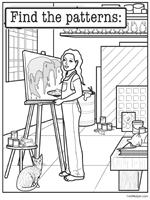Finding Patterns or Groups of Shapes Together

Even and Odd Numbers
Circle odd or even (numbers 1 to 29)
Circle odd or even (numbers 1 to 99)
Write odd or even

Before, After, Between
Just before and after, Just before, Just after
Even and odd numbers: numbers 3 to 29 (easier)
Even and odd numbers: numbers 3 to 29
Even and odd numbers: numbers 10 to 99 (easier)
Even and odd numbers: numbers 10 to 99

Counting
Counting forward and backward (with a number line)
Counting forward and backward

Skip-counting (includes hint on how to skip count)
Skip count by 2's (numbers 2 to 49)
Skip count by 3's (numbers 2 to 49)
Skip count by 4's (numbers 2 to 49)
Skip count by 5's (numbers 2 to 49)
Skip count by 2's, 3's, 4's, and 5's (numbers 2 to 49)
Skip count by 2's (numbers 2 to 99)
Skip count by 3's (numbers 2 to 99)
Skip count by 4's (numbers 2 to 99)
Skip count by 5's (numbers 2 to 99)
Skip count by 2's, 3's, 4's, and 5's (numbers 2 to 99)
Mix of skip count with larger problems and more blanks

Skip-counting
Mix of complete the pattern (numbers 2 to 49)
Mix of complete the pattern (numbers 2 to 99)
Mix of complete the pattern (numbers 2 to 99 with more blanks per question)

Repeating Patterns
One blank at end (numbers 1-20)
One blank at end (numbers 1-99)
Two to three blanks at end (numbers 1-20)
Two to three blanks at end (numbers 1-99)
One blank anywhere in the pattern (numbers 1-99)

Problem Solving
Problem solving

Review
Number Patterns Mixed Review

Sequences Math Puzzles
The student must figure out what pattern is being used.
Multiple lines using the same pattern are given.
Positive Whole Numbers
Positive Whole Numbers
Positive and Negative Whole Numbers
Decimals

Teacher Recommendations:  Worksheet and Lesson Plan Activity Ideas

Math

Money

Science

Word Search

Writing

Shapes

Cause And Effect

Spelling

Solar System

Time